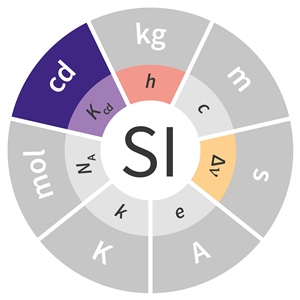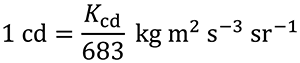# SI base unit: candela (cd)

The candela, symbol cd, is the SI unit of luminous intensity in a given direction. It is defined by taking the fixed numerical value of the luminous efficacy of monochromatic radiation of frequency 540 x 1012 Hz, Kcd, to be 683 when expressed in the unit lm W–1, which is equal to cd sr W–1, or cd sr kg–1 m–2 s3, where the kilogram, metre and second are defined in terms of h, c and ΔνCs.This definition implies the exact relation Kcd = 683 cd sr kg–1 m–2 s3 for monochromatic radiation of frequency ν = 540 x 1012 Hz. Inverting this relation gives an exact expression for the candela in terms of the defining constants Kcd, h and ΔνCs:which is equal toThe effect of this definition is that one candela is the luminous intensity, in a given direction, of a source that emits monochromatic radiation of frequency 540 x 1012 Hz and has a radiant intensity in that direction of (1/683) W/sr.